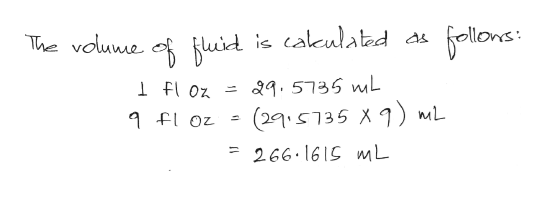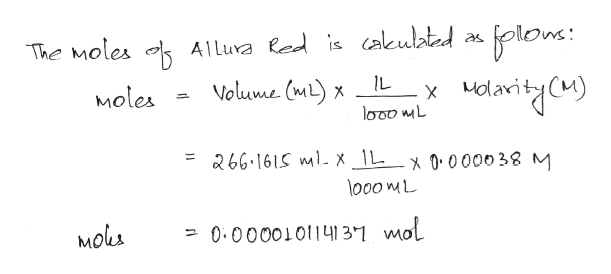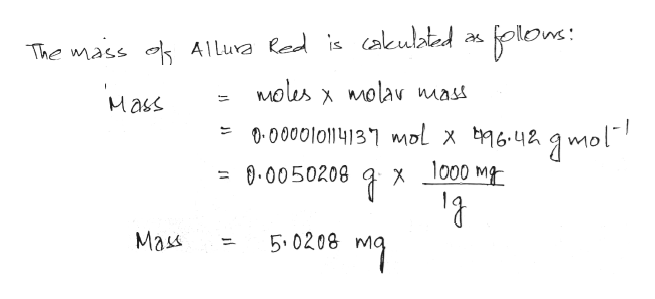# Many common materials that we ingest, though quite safe in reasonable quantities, become toxic when taken in very large doses. A measure of toxicity is the LD50 value (Lethal Dose, 50%). It is the quantity of material, expressed in mg of material per kg of subject-body-weight that, if administered to a population of subjects, would cause 50% of the population to die. The LD50 value for FD&C Red Dye No. 40 is >10,000 mg/kg in rats. Assume that the LD50 value for humans is the same as for rats.Calculate the number of mg of Allura Red present in an 9 fluid ounce glass of the beverage you used in this lab and use this calculation to determine the number of such glasses of Allura Red sports drink required to reach the LD50 of 10,000 mg of Allura Red/kg body weight. Assume that the concentration of Allura Red in the beverage is 0.000038 M and the person has a body weight of 175 lbs.The molar mass of Allura Red is 496.42 grams/mol1 fl oz = 29.5735 mL2.205 lbs = 1 kgYou may assume two significant figures but do not use scientific notation. Do not include units with your answer.

Question
1 views

Many common materials that we ingest, though quite safe in reasonable quantities, become toxic when taken in very large doses. A measure of toxicity is the LD50 value (Lethal Dose, 50%). It is the quantity of material, expressed in mg of material per kg of subject-body-weight that, if administered to a population of subjects, would cause 50% of the population to die. The LD50 value for FD&C Red Dye No. 40 is >10,000 mg/kg in rats. Assume that the LD50 value for humans is the same as for rats.

Calculate the number of mg of Allura Red present in an 9 fluid ounce glass of the beverage you used in this lab and use this calculation to determine the number of such glasses of Allura Red sports drink required to reach the LD50 of 10,000 mg of Allura Red/kg body weight. Assume that the concentration of Allura Red in the beverage is 0.000038 M and the person has a body weight of 175 lbs.

The molar mass of Allura Red is 496.42 grams/mol

1 fl oz = 29.5735 mL

2.205 lbs = 1 kg

You may assume two significant figures but do not use scientific notation.

check_circle

Step 1help_outlineImage TranscriptioncloseThe vouwe 6llowes fuid is cakculated as 29.5735 mL Foz (29S735 X) mL floz 266.1615 mL fullscreen
Step 2help_outlineImage Transcriptioncloseplon: The Moles Alluva led is cakulated Volume (m)x IL Moles 266.161S mlX \L_x 0000o38 M lo00 mL Mols -0.0000101141 3-1 mol fullscreen
Step 3help_outlineImage TranscriptioncloseThe mass Alluva led is cakulated alons Molus molav mass X Mass 1-0000l011413 l x 642mol 000 M 0.0050208 X Mass 5.0208 fullscreen

### Want to see the full answer?

See Solution

#### Want to see this answer and more?

Solutions are written by subject experts who are available 24/7. Questions are typically answered within 1 hour.*

See Solution
*Response times may vary by subject and question.
Tagged in

### General Chemistry